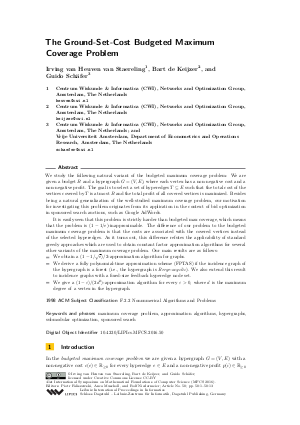Document# The Ground-Set-Cost Budgeted Maximum Coverage Problem

### Authors Irving van Heuven van Staereling, Bart de Keijzer, Guido Schäfer## File

LIPIcs.MFCS.2016.50.pdf
• Filesize: 492 kB
• 13 pages

## Cite As

Irving van Heuven van Staereling, Bart de Keijzer, and Guido Schäfer. The Ground-Set-Cost Budgeted Maximum Coverage Problem. In 41st International Symposium on Mathematical Foundations of Computer Science (MFCS 2016). Leibniz International Proceedings in Informatics (LIPIcs), Volume 58, pp. 50:1-50:13, Schloss Dagstuhl - Leibniz-Zentrum für Informatik (2016)
https://doi.org/10.4230/LIPIcs.MFCS.2016.50

## Abstract

We study the following natural variant of the budgeted maximum coverage problem: We are given a budget B and a hypergraph G = (V, E), where each vertex has a non-negative cost and a non-negative profit. The goal is to select a set of hyperedges T subseteq E such that the total cost of the vertices covered by T is at most B and the total profit of all covered vertices is maximized. Besides being a natural generalization of the well-studied maximum coverage problem, our motivation for investigating this problem originates from its application in the context of bid optimization in sponsored search auctions, such as Google AdWords. It is easily seen that this problem is strictly harder than budgeted max coverage, which means that the problem is (1-1/e)-inapproximable. The difference of our problem to the budgeted maximum coverage problem is that the costs are associated with the covered vertices instead of the selected hyperedges. As it turns out, this difference refutes the applicability of standard greedy approaches which are used to obtain constant factor approximation algorithms for several other variants of the maximum coverage problem. Our main results are as follows: - We obtain a (1 - 1/sqrt(e))/2-approximation algorithm for graphs. - We derive a fully polynomial-time approximation scheme (FPTAS) if the incidence graph of the hypergraph is a forest (i.e., the hypergraph is Berge-acyclic). We also extend this result to incidence graphs with a fixed-size feedback hyperedge node set. - We give a (1-epsilon)/(2d^2)-approximation algorithm for every epsilon > 0, where d is the maximum degree of a vertex in the hypergraph.
##### Keywords
• maximum coverage problem
• approximation algorithms
• hypergraphs
• submodular optimization

## Metrics

• Access Statistics
• Total Accesses (updated on a weekly basis)
0

## References

1. G. Calinescu, C. Chekuri, M. Pál, and J. Vondrák. Maximizing a monotone submodular function subject to a matroid constraint. SIAM Journal on Computing, 40(6):1740-1766, 2011.2. Y. Cao, J. Chen, and Y. Liu. On feedback vertex set new measure and new structures. In Proceedings of the 12th Scandinavian conference on Algorithm Theory, pages 93-104. Springer-Verlag, 2010.3. R. Cohen and L. Katzir. The generalized maximum coverage problem. Information Processing Letters, 108(1):15-22, 2008.4. U. Feige. A threshold of ln n for approximating set cover. Journal of the ACM, 45(4):634-652, 1998.5. J. Feldman, S. Muthukrishnan, M. Pál, and C. Stein. Budget optimization in search-based advertising auctions. In Proceedings of the 8th ACM conference on electronic commerce, pages 40-49, New York, NY, USA, 2007. ACM.6. D.S. Hochbaum, editor. Approximation Algorithms for NP-hard Problems. PWS Publishing Co., 1997.7. R.K. Iyer and J.A. Bilmes. Submodular optimization with submodular cover and submodular knapsack constraints. In Advances in Neural Information Processing Systems, pages 2436-2444. MIT Press, 2013.8. H. Kellerer, U. Pferschy, and D. Pisinger. Knapsack Problems. Springer-Verlag, 2004.9. S. Khuller, A. Moss, and J. Naor. The budgeted maximum coverage problem. Information Processing Letters, 70(1):39-45, 1999.10. Z. Svitkina and L. Fleischer. Submodular approximation: Sampling-based algorithms and lower bounds. SIAM Journal of Computing, 40(6):1715-1737, 2011.11. V.V. Vazirani. Approximation algorithms. Springer-Verlag, 2003.X

Feedback for Dagstuhl Publishing